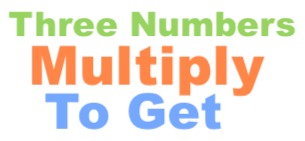What three numbers multiply to get 60?What three numbers multiply to get 60? In other words, you want to know all the combinations of 3 numbers that can be multiplied to get 60. You want to know what all the question marks in the equation below can be in order for the equation to be true:

? × ? × ? = 60

First, note that all numbers must be 60 or less, which means that there are 60 × 60 × 60 possible solutions. Thus, to get the answer to "What three numbers multiply to get 60?" we looked at 216,000 different combinations to see which ones equal 60.

When we looked at all those combinations, we found 54 different combinations of 3 numbers that equal 60 when multiplied together. Here are all 3 number combinations that you can multiply to get 60:

60 × 1 × 1 = 60
30 × 2 × 1 = 60
30 × 1 × 2 = 60
20 × 3 × 1 = 60
20 × 1 × 3 = 60
15 × 4 × 1 = 60
15 × 2 × 2 = 60
15 × 1 × 4 = 60
12 × 5 × 1 = 60
12 × 1 × 5 = 60
10 × 6 × 1 = 60
10 × 3 × 2 = 60
10 × 2 × 3 = 60
10 × 1 × 6 = 60
6 × 10 × 1 = 60
6 × 5 × 2 = 60
6 × 2 × 5 = 60
6 × 1 × 10 = 60
5 × 12 × 1 = 60
5 × 6 × 2 = 60
5 × 4 × 3 = 60
5 × 3 × 4 = 60
5 × 2 × 6 = 60
5 × 1 × 12 = 60
4 × 15 × 1 = 60
4 × 5 × 3 = 60
4 × 3 × 5 = 60
4 × 1 × 15 = 60
3 × 20 × 1 = 60
3 × 10 × 2 = 60
3 × 5 × 4 = 60
3 × 4 × 5 = 60
3 × 2 × 10 = 60
3 × 1 × 20 = 60
2 × 30 × 1 = 60
2 × 15 × 2 = 60
2 × 10 × 3 = 60
2 × 6 × 5 = 60
2 × 5 × 6 = 60
2 × 3 × 10 = 60
2 × 2 × 15 = 60
2 × 1 × 30 = 60
1 × 60 × 1 = 60
1 × 30 × 2 = 60
1 × 20 × 3 = 60
1 × 15 × 4 = 60
1 × 12 × 5 = 60
1 × 10 × 6 = 60
1 × 6 × 10 = 60
1 × 5 × 12 = 60
1 × 4 × 15 = 60
1 × 3 × 20 = 60
1 × 2 × 30 = 60
1 × 1 × 60 = 60

Three Numbers Multiply to Get Calculator
Do you need a different combination of three numbers that when multiplied equal a number other than 60? No problem! Please enter your number here.

What three numbers multiply to get 61?
Here is the next problem on our list that we have explained and answered for your convenience.